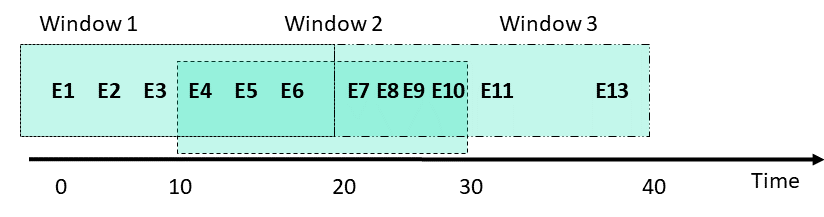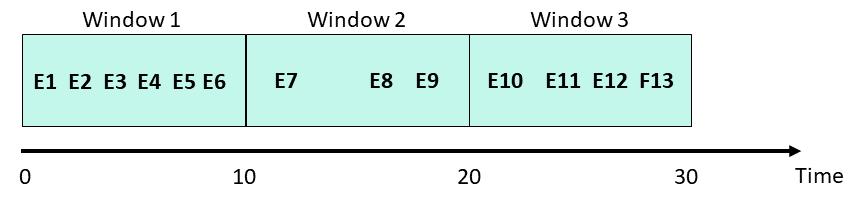# Feature set transformations#

A feature set contains an execution graph of operations that are performed when data is ingested, or when simulating data flow for inferring its metadata. This graph utilizes MLRun’s Real-time serving pipelines (graphs).

The graph contains steps that represent data sources and targets, and may also contain steps whose purpose is transformations and enrichment of the data passed through the feature set. These transformations can be provided in one of three ways:

Once a feature-set is created, its internal execution graph can be observed by calling the feature-set’s `plot()` function, which generates a `graphviz` plot based on the internal graph. This is very useful when running within a Jupyter notebook, and produces a graph such as the following example:

This plot shows various transformations and aggregations being used as part of the feature-set processing, as well as the targets where results are saved to (in this case two targets). Feature-sets can also be observed in the MLRun UI, where the full graph can be seen and specific step properties can be observed:

For a full end-to-end example of feature-store and usage of the functionality described in this page, refer to the feature store example.

In this section

## Aggregations#

Aggregations, being a common tool in data preparation and ML feature engineering, are available directly through the MLRun `FeatureSet` class. These transformations add a new feature to the feature-set that is created by performing an aggregate function over the feature’s values. You can use aggregation for time-based sliding windows and fixed windows. In general, sliding windows are used for real time data, while fixed windows are used for historical aggregations.

A window can be measured in years, days, hours, seconds, minutes. A window can be a single window, e.g. ‘1h’, ‘1d’, or a list of same unit windows e.g. [‘1h’, ‘6h’]. If you define the time period (in addition to the window), then you have a sliding window. If you don’t define the time period, then the time period and the window are the same. All time windows are aligned to the epoch (1970-01-01T00:00:00Z).

• Sliding window

Sliding windows are fixed-size, overlapping, windows (defined by `windows`) that are evaluated at a sliding interval (defined by `period`).
The period size must be an integral divisor of the window size.

The following figure illustrates sliding windows of size 20 seconds, and periods of 10 seconds. Since the period is less than the window size, the windows contain overlapping data. In this example, events E4-E6 are in Windows 1 and 2. When Window 2 is evaluated at time t = 30 seconds, events E4-E6 are dropped from the event queue.The following code illustrates a feature-set that contains stock trading data including the specific bid price for each bid at any given time. You can add aggregate features that show the minimal and maximal bidding price over all the bids in the last 60 minutes, evaluated (sliding) at a 10 minute interval, per stock ticker (which is the entity in question).

```import mlrun.feature_store as fstore
# create a new feature set
quotes_set = fstore.FeatureSet("stock-quotes", entities=[fstore.Entity("ticker")])
quotes_set.add_aggregation("bid", ["min", "max"], ["1h"], "10m", name="price")
```

This code generates two new features: `bid_min_1h` and `bid_max_1h` every 10 minutes.

• Fixed window

A fixed window has a fixed-size, is non-overlapping, and gapless. A fixed time window is used for aggregating over a time period, (or day of the week). For example, how busy is a restaurant between 1 and 2 pm.
When using a fixed window, each record in an in-application stream belongs to a specific window. The record is processed only once (when the query processes the window to which the record belongs).To define a fixed window, omit the time period. Using the above example, but for a fixed window:

```import mlrun.feature_store as fstore
# create a new feature set
quotes_set = fstore.FeatureSet("stock-quotes", entities=[fstore.Entity("ticker")])
```

This code generates two new features: `bid_min_1h` and `bid_max_1h` once per hour.

If the `name` parameter is not specified, features are generated in the format `{column_name}_{operation}_{window}`.
If you supply the optional `name` parameter, features are generated in the format `{name}_{operation}_{window}`.

These features can be fed into predictive models or be used for additional processing and feature generation.

Notes

• Internally, the graph step that is created to perform these aggregations is named `"Aggregates"`. If more than one aggregation steps are needed, a unique name must be provided to each, using the `state_name` parameter.

• The timestamp column must be part of the feature set definition (for aggregation).

Aggregations that are supported using this function are:

• `count`

• `sum`

• `sqr` (sum of squares)

• `max`

• `min`

• `first`

• `last`

• `avg`

• `stdvar`

• `stddev`

For a full documentation of this function, see the `add_aggregation()` documentation.

## Built-in transformations#

MLRun, and the associated `storey` package, have a built-in library of transformation functions that can be applied as steps in the feature-set’s internal execution graph. To add steps to the graph,
reference them from the `FeatureSet` object by using the `graph` property. Then, new steps can be added to the graph using the functions in `storey.transformations` (follow the link to browse the documentation and the list of existing functions). The transformations are also accessible directly from the `storey` module.

See the built-in steps.

Note

Internally, MLRun makes use of functions defined in the `storey` package for various purposes. When creating a feature-set and configuring it with sources and targets, what MLRun does behind the scenes is to add steps to the execution graph that wraps methods and classes that perform the actions. When defining an async execution graph, `storey` classes are used. For example, when defining a Parquet data-target in MLRun, a graph step is created that wraps storey’s ParquetTarget function.

To use a function:

1. Access the graph from the feature-set object, using the `graph` property.

2. Add steps to the graph using the various graph functions, such as `to()`. The function object passed to the step should point at the transformation function being used.

The following is an example for adding a simple `filter` to the graph, that drops any bid that is lower than 50USD:

```quotes_set.graph.to("storey.Filter", "filter", _fn="(event['bid'] > 50)")
```

In the example above, the parameter `_fn` denotes a callable expression that is passed to the `storey.Filter` class as the parameter `fn`. The callable parameter can also be a Python function, in which case there’s no need for parentheses around it. This call generates a step in the graph called `filter` that calls the expression provided with the event being propagated through the graph as the data is fed to the feature-set.

## Custom transformations#

When a transformation is needed that is not provided by the built-in functions, new classes that implement transformations can be created and added to the execution graph. Such classes should extend the `MapClass` class, and the actual transformation should be implemented within their `do()` function, which receives an event and returns the event after performing transformations and manipulations on it. For example, consider the following code:

```class MyMap(MapClass):
def __init__(self, multiplier=1, **kwargs):
super().__init__(**kwargs)
self._multiplier = multiplier

def do(self, event):
event["multi"] = event["bid"] * self._multiplier
return event
```

The `MyMap` class can then be used to construct graph steps, in the same way as shown above for built-in functions:

```quotes_set.graph.add_step("MyMap", "multi", after="filter", multiplier=3)
```

This uses the `add_step` function of the graph to add a step called `multi` utilizing `MyMap` after the `filter` step that was added previously. The class is initialized with a multiplier of 3.